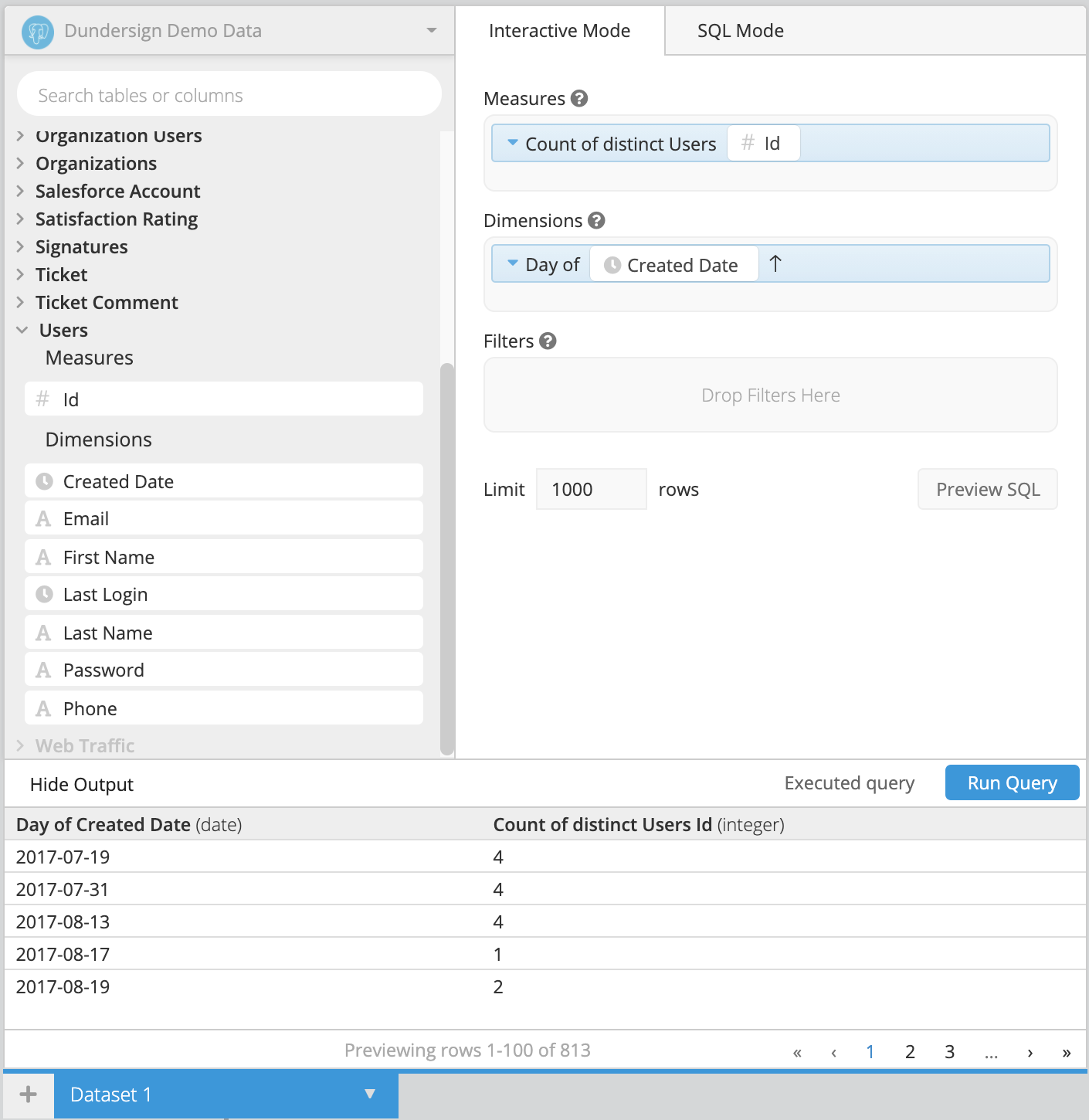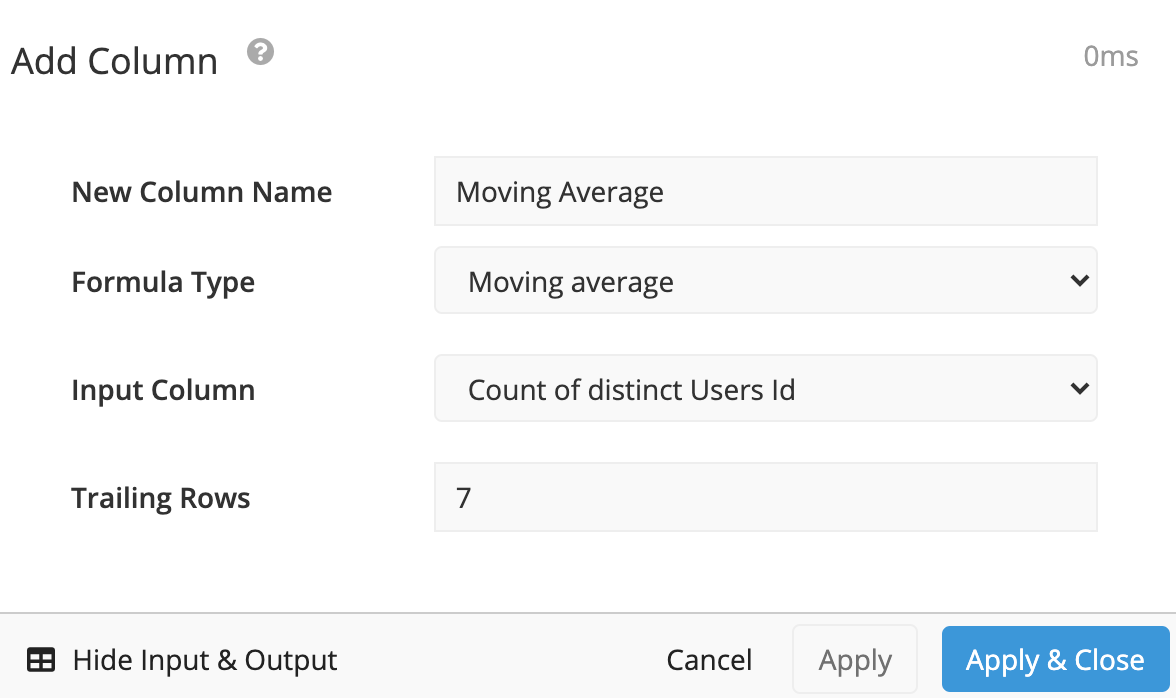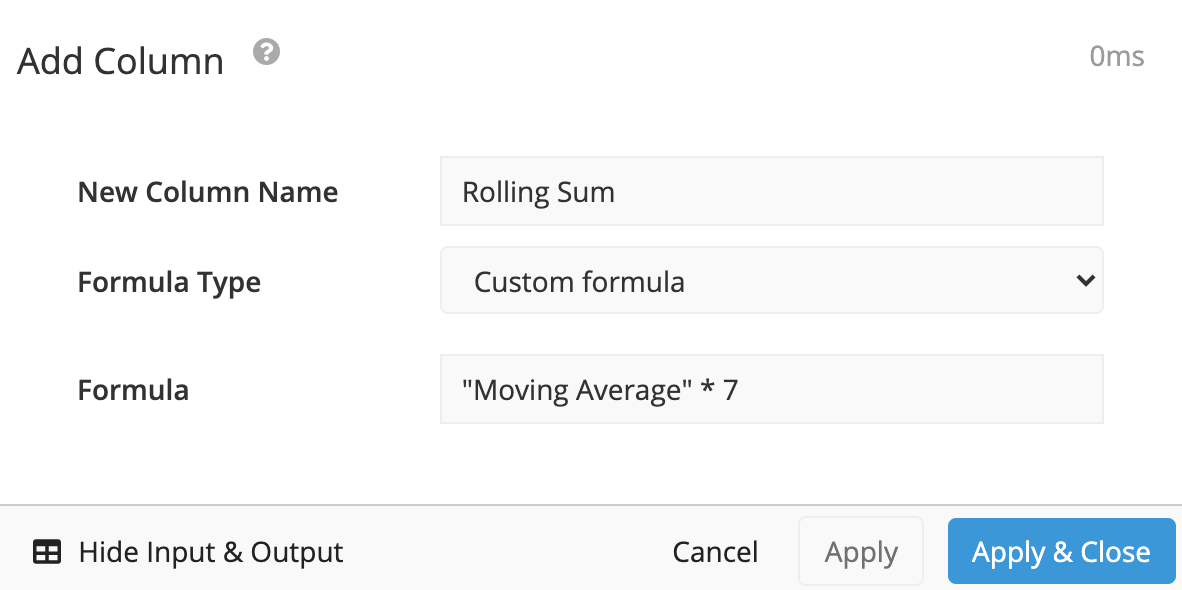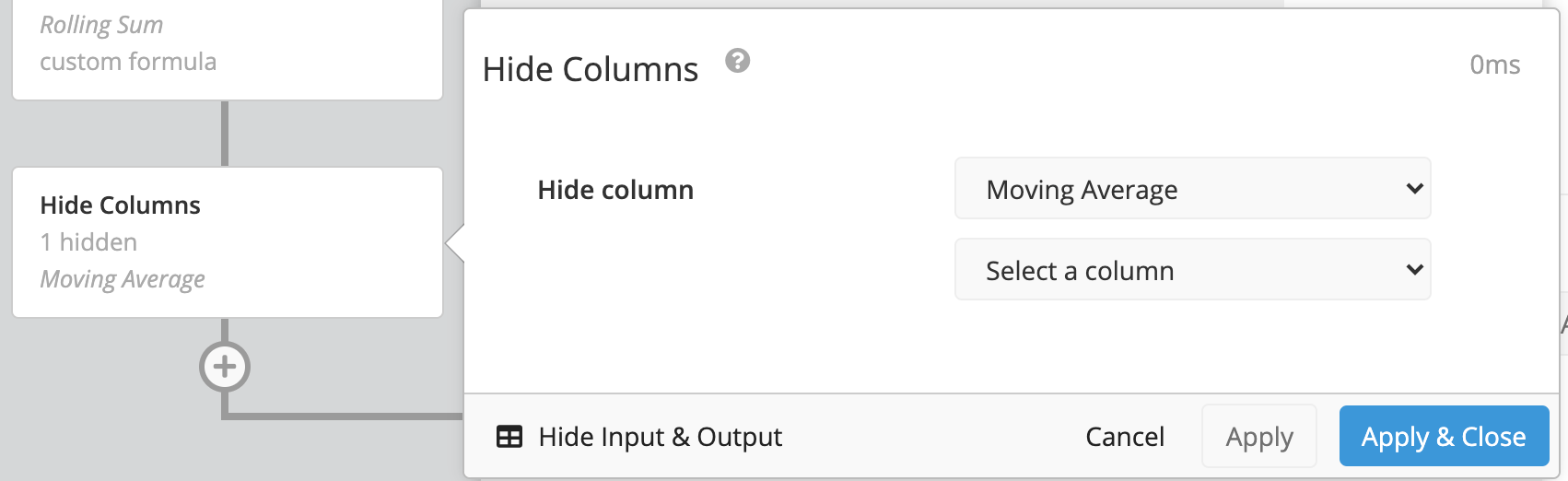# Calculate a rolling sum in Data Explorer

You can calculate a moving average in Chartio by using our Moving Average pre-built formula in the Data Pipeline using an Add Column or Edit Column Pipeline step. In the event you’d like to calculate a rolling sum (or moving sum), you can easily edit the Moving Average to multiply it by the selected period.

In the example below, we create a chart that pulls the count of users per day. Here, we also want to include a column that calculates the rolling sum of the count of users in the last seven days. This is different from a standard running total because it only includes the sum of the previous seven rows of data.

To do this, follow the steps below:

1. Start by building a chart for the count of users per day.

Here, we use the Users table from our Dundersign Demo Data data source. We drag the Id column into the Measures field and the Day of Created Date into the Dimensions field. Click Run Query.2. Add a new column in the Data Pipeline to calculate the moving average.

Click +Add Transformation below your Dataset query and select Add Column from the options. Select Moving average for the Formula Type and Count of distinct Users User Id for the Input Column. Enter 7 for the Trailing Rows; this ensure you only calculate the moving average for the last seven days. Click Apply & Close when you’re done.

Note: If you don’t have rows for every day and want to make sure you calculate null values for the last week, add a Zero Fill Data Pipeline step before the Add Column step.1. The moving average is simply the sum of the last seven values divided by 7. Therefore, we can get our rolling sum by multiplying the moving average by seven.

Use another Add Column step to do exactly that. Simply select Custom formula for the Formula Type and use the following formula, replacing `Moving Average` with your custom column from Step 2.

`````` "Moving Average" * 7
``````2. Since it’s no longer necessary, add a Hide Columns step to hide the Moving average column. Click Apply & Close.3. Switch your chart to a Line chart from the chart types to visualize your daily user count compared to the rolling user sum. Don’t forget to save your chart to your dashboard when done.